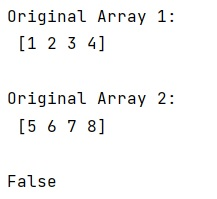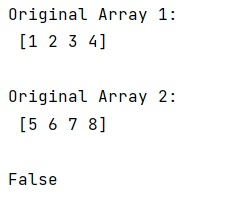# Use numpy's any() and all() methods

Learn, how to use numpy's any() and all() methods in Python?
Submitted by Pranit Sharma, on January 22, 2023

NumPy is an abbreviated form of Numerical Python. It is used for different types of scientific operations in python. Numpy is a vast library in python which is used for almost every kind of scientific or mathematical operation. It is itself an array which is a collection of various methods and functions for processing the arrays.

## numpy.any() Method

The numpy.any() method is used to test whether any array element along a given axis evaluates to True and returns a single boolean if the axis is None.

Syntax:

```numpy.any(
a,
axis=None,
out=None,
keepdims=<no value>,
*,
where=<no value>
)
```

## Python code to demonstrate how to use numpy.any() method

```# Import numpy
import numpy as np

# Creating two numpy arrays
arr1 = np.array([1,2,3,4])
arr2 = np.array([5,6,7,8])

# Display original arrays
print("Original Array 1:\n",arr1,"\n")
print("Original Array 2:\n",arr2,"\n")

# Check with any
print((arr1 == arr2 ).any())
```

Output:## numpy.all() Method

The numpy.all() method is used to test whether all array elements along a given axis evaluate to True and returns a new boolean or array is returned unless out is specified, in which case a reference to out is returned.

Syntax:

```numpy.all(
a,
axis=None,
out=None,
keepdims=<no value>,
*,
where=<no value>
)
```

## Python code to demonstrate how to use numpy.all() method

```# Import numpy
import numpy as np

# Creating two numpy arrays
arr1 = np.array([1,2,3,4])
arr2 = np.array([5,6,7,8])

# Display original arrays
print("Original Array 1:\n",arr1,"\n")
print("Original Array 2:\n",arr2,"\n")

# Check with all
print((arr1 == arr2 ).all())
```

Output:What's New (MCQs)

Top Interview Coding Problems/Challenges!

IncludeHelp's Blogs

Languages: » C » C++ » C++ STL » Java » Data Structure » C#.Net » Android » Kotlin » SQL
Web Technologies: » PHP » Python » JavaScript » CSS » Ajax » Node.js » Web programming/HTML
Solved programs: » C » C++ » DS » Java » C#
Aptitude que. & ans.: » C » C++ » Java » DBMS
Interview que. & ans.: » C » Embedded C » Java » SEO » HR
CS Subjects: » CS Basics » O.S. » Networks » DBMS » Embedded Systems » Cloud Computing
» Machine learning » CS Organizations » Linux » DOS
More: » Articles » Puzzles » News/Updates